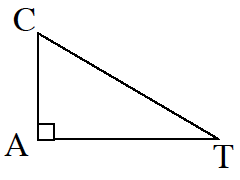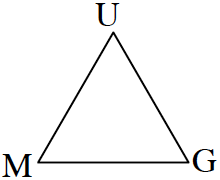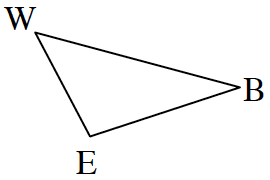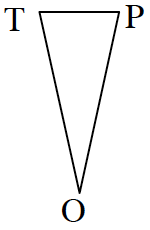### Home > MC2 > Chapter 9 > Lesson 9.2.3 > Problem9-65

9-65.

If each triangle below is drawn accurately, identify whether it is most likely an obtuse, acute, or right triangle. Label the angle or angles that helped you to decide.

1.Examine all three angles of this figure.
Are the measures of any of the angles greater than or equal to $90°$?

The box on angle A indicates that angle A is a right angle.
The figure is therefore identified as a right triangle.

1.See part (a).

Can you explain why this is an acute triangle?

1.See part (a).

This figure is an obtuse triangle because the measure of one of its angles is greater than $90°$.

1.See part (a).